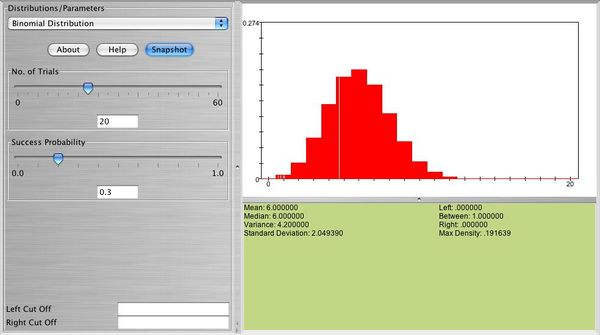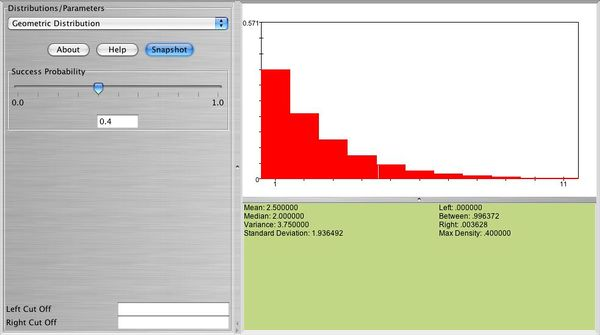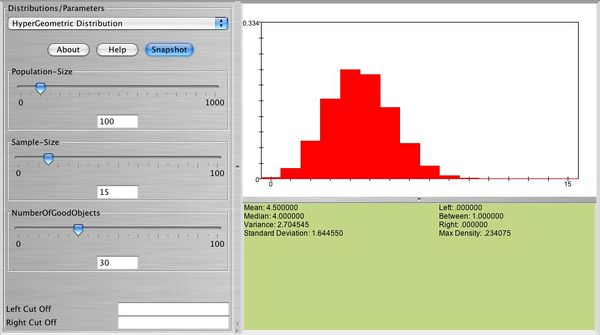# SOCR EduMaterials Activities Binomial Distributions

## This is an activity to explore the Binomial, Geometric, and Hypergeometric Probability Distributions.

• Exercise 1: Use SOCR to graph and print the following distributions and answer the questions below. Also, comment on the shape of each one of these distributions:
• a. \( X \sim b(10,0.5) \), find \( P(X=3) \), \( E(X) \), \( sd(X) \), and verify them with the formulas discussed in class.
• b. \( X \sim b(10,0.1) \), find \( P(1 \le X \le 3) \).
• c. \( X \sim b(10,0.9) \), find \( P(5 < X < 8), \ P(X < 8), \ P(X \le 7), \ P(X \ge 9) \).
• d. \( X \sim b(30,0.1) \), find \( P(X > 2) \).

Below you can see a snapshot of the distribution of \( X \sim b(20,0.3) \)• Exercise 2: Use SOCR to graph and print the distribution of a geometric random variable with \( p=0.2, p=0.7 \). What is the shape of these distributions? What happens when \( p \) is large? What happens when \( p \) is small?

Below you can see a snapshot of the distribution of \( X \sim geometric(0.4) \)• Exercise 3: Select the geometric probability distribution with \( p=0.2 \). Use SOCR to compute the following:
• a. \( P(X=5) \)
• b. \( P(X > 3) \)
• c. \( P(X \le 5) \)
• d. \( P(X > 6) \)
• e. \( P(X \ge 8) \)
• f. \( P(4 \le X \le 9) \)
• g. \( P(4 < X < 9) \)
• Exercise 4: Verify that your answers in exercise 3 agree with the formulas discussed in class, for example, \( P(X=x)=(1-p)^{x-1}p \), \( P(X > k)=(1-p)^k \), etc. Write all your answers in detail using those formulas.
• Exercise 5: Let \( X \) follow the hypergeometric probability distribution with \( N=52 \), \( n=10 \), and number of "hot" items 13. Use SOCR to graph and print this distribution.

Below you can see a snapshot of the distribution of \( X \sim hypergeometric(N=100, n=15, r=30) \)• Exercise 6: Refer to exercise 5. Use SOCR to compute \( P(X=5) \) and write down the formula that gives this answer.
• Exercise 7: Binomial approximation to hypergeometric: Let \( X \) follow the hypergeometric probability distribution with \( N=1000, \ n=10 \) and number of "hot" items 50. Graph and print this distribution.
• Exercise 8: Refer to exercise 7. Use SOCR to compute the exact probability\[ P(X=2) \]. Approximate \( P(X=2) \) using the binomial distribution. Is the approximation good? Why?
• Exercise 9: Do you think you can approximate well the hypergeometric probability distribution with \( N=50, \ n=20 \), and number of "hot" items 40 using the binomial probability distribution? Graph and print the exact (hypergeometric) and the approximate (binomial) distributions and compare.